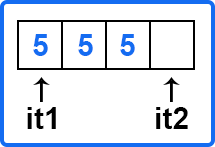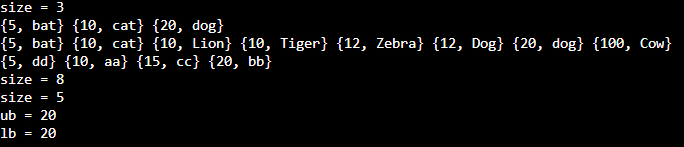# MultiMap in C++

## MultiMap in C++ with Examples:

In this article, I am going to discuss MultiMap in C++ with Examples. Please read our previous article where we discussed Map in C++ with Examples.

##### MultiMap in C++ with Examples:

In the last article, we had seen a map data structure and we had seen that it keeps a unique pair of keys and values. So here the name says multi-map you can have multiple keys. So duplicate keys are allowed. Most of the things will be similar to a map but a big difference is that multiple keys are allowed with the same value. So, for example, if 10 is mapped to ‘a’ and we go ahead and insert 10 again with a value of ‘b’ then this is allowed in multi-map but in the case of the map only the first occurrence will be there this will not be inserted at all. Multimap is present in the ‘map’ header file. Multimap is usually implemented as RB trees,It keeps the element in sorted order. Users can provide a custom compare function to change the ordering and here also its underlying data structure is a binary search tree and in most of the implementation, it is a red-black tree. Although it is not specified in the standard that which data structure exactly to use. Search, removal, and insertion takes logarithmic times. Now let us see some of the functions.

##### MultiMap Functions in C++:
1. Size () – This function is used to find the number of elements present in the multimap container. It does not return the unique keys since duplicate keys are also inserted.
2. Equal (=) – it is used to assign multimap.
3. Clear () – it will remove all the elements from the multimap container.
4. Count (), find () – In the case of the map, it will return 0 or 1 by checking whether the key is present or not. In multimap, it will return the actual number of values that are mapped to the same key. For example, if we have 10 a, and 10 b then count 10 will return 2 because there are 2 keys with a value of 10. Find will return one of the elements that are matching to the parameter that you pass in the function. We will see an example of this. If 10 is present, it will return one of these 10. It is not guaranteed which 10 will be accessed
5. Contains (key) – It is present in C++20. It is of Boolean type. It will check the presence of the given key.
6. Empty () – it will check and return whether the multimap is empty or not.
7. upper_bound (key), lower_bound (key) – Lower bound is ‘>=’ and upper bound is ‘<’. So, let us say we have keys 10, 10, 15, and 20 associated with some values and these will be sorted. So, 10 will come before 15 then we have some values having key 20 and if we call lower_bound (15) so it means greater than or equal to 15. It will return an iterator to this point and the upper bound means greater than so it will return 20 because it cannot return 15. It will return more than 20. So, it will return an iterator to this one upper bound and lower bound. Let us say we are calling a lower bound on some value 17. So greater than or equal to 17 20 so the lower bound will return 20 and the upper bound will also return 20. So, these can be equal or may not be equal depending on the data that we are providing.
8. Equal_range (key) – This function was not present in the map. In multimap, we can have multiple entries of the same key so let us say we have three entries of 5 and we call equal_range (5). So, we have some values before and after 5 and these are sorted. It will return the following pair of iterators it1 and it2.So, using this you can access all the tens.

Insert () and erase () – we will see these functions in detail.

In the map, we can use [] or at the operator to get the value at that particular position but in multimap, [] and at operator are not defined. So, we cannot use them.

##### Insert MultiMap Functions in C++:
1. Insert (Key key): It may not inset always. Let’s say we have a set, s = {1, 3, 2, 3, 6, 1}. Here, 1 is already present so insert (Key key) will not insert 1 there. Now suppose we have a multimap, m = {1, 2, 3, 4, 4, 4, 5} and we want to insert 4 so where it will insert 4? It will insert at the upper bound. The Upper bound for this is 5 so it will insert 4 before 5. Now m = {1, 2, 3, 4, 4, 4, 4, 5}.
2. Insert (iterator pos1, iterator pos2): Here, we can pass two iterator positions. Let us say you have a vector of elements, V = {_ _ _ x _ _ _ y _ _ _ _ } You want to insert from position x to position y (excluding y) and these are iterator positions. It is like range [pos1, pos2). So, the close interval at pos1 and an open interval at pos2. This function will not return anything.
3. Insert (initializer_list l): Similarly, we can pass the initializer list. You can think of it as in the above example if we are inserting from pos1 to pos2, so it will be an initializer list or you can say a subset of a vector. So, you will pass that initializer list and it will add all the elements to the multimap. It will also not return anything. We will see examples of all of these functions.
##### Erase MultiMap Functions in C++:
1. erase (iterator pos): It removes the element at the given position and returns the iterator following the last element removed.
2. erase (iterator pos1, iterator pos2): As we have seen in the insert function, we can also pass two positions in this function so that all the elements between these positions will be removed. So pos1 will be included and pos2 will be excluded. It also returns the iterator following the last element removed.
3. erase (key): In this signature, we have passed the iterator to the key but here we are passing the key itself. If any key is present in the multimap, it will remove all the elements with the key values. It will return the number of elements removed.

Now let’s see the complete program.

##### Example to understand Multimap in C++:
```#include <iostream>
#include <map>
using namespace std;

int main() {
multimap<int, string> m_map = {{10, "cat"}, {20, "dog"}, {5, "bat"}};
cout << "size = " << m_map.size() << endl;

for(auto& p: m_map)
cout << "{" << p.first << ", " << p.second << "} ";
cout << endl;

m_map.insert({100, "Cow"});
m_map.insert({10, "Lion"});
m_map.insert({{10, "Tiger"}, {12, "Zebra"}});
m_map.insert(make_pair<int, string>(12, "Dog"));

for(auto& p: m_map)
cout << "{" << p.first << ", " << p.second << "} ";
cout << endl;

map<int, string> m_map2 = {{10, "aa"}, {20, "bb"}, {15, "cc"}, {5, "dd"}};
m_map2.insert(m_map2.begin(), m_map2.end());

for(auto& p: m_map2)
cout << "{" << p.first << ", " << p.second << "} ";
cout << endl;

cout << "size = " << m_map.size() << endl;
auto it = m_map.erase(10);//
//m.erase(m.find(10));
cout << "size = " << m_map.size() << endl;

auto ub = m_map.upper_bound(15);
auto lb = m_map.lower_bound(15);
cout << "ub = " << ub->first << endl;
cout << "lb = " << lb->first << endl;

auto range = m_map.equal_range(10);
for(auto it = range.first; it != range.second; ++it)
cout << it->second << "   ";
cout << endl;
}
```
###### Output: